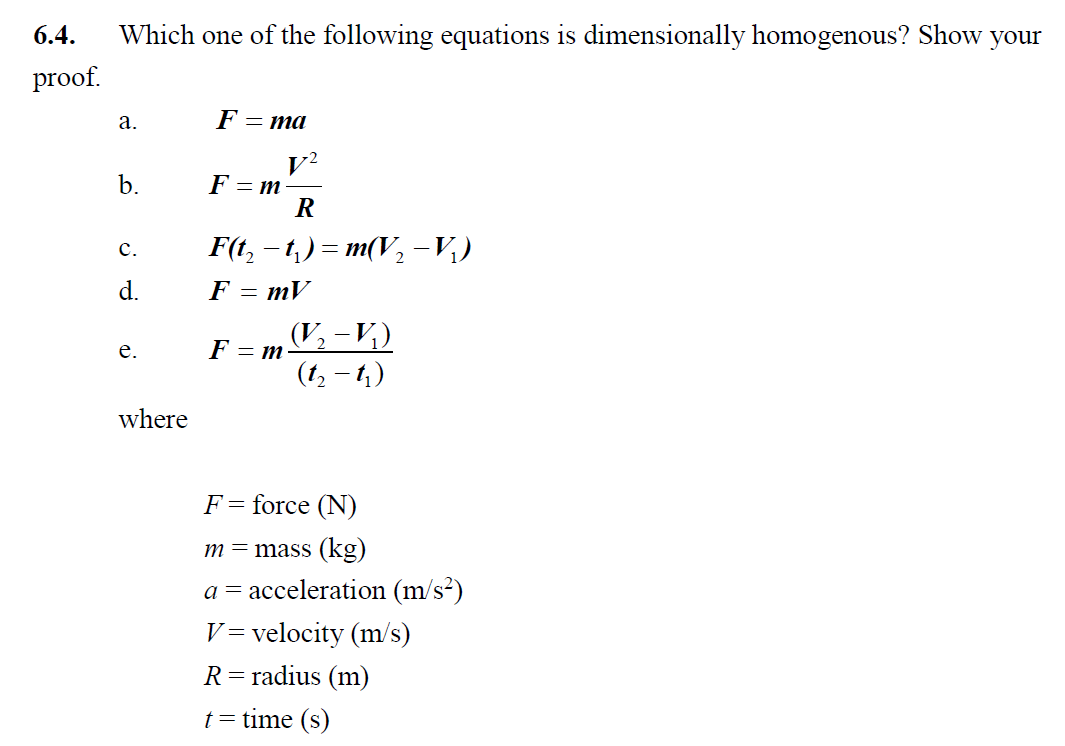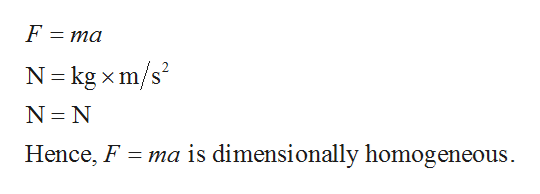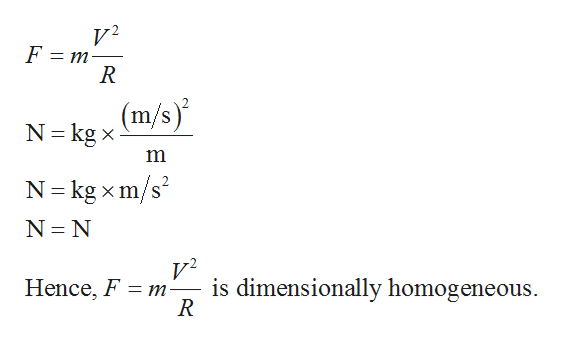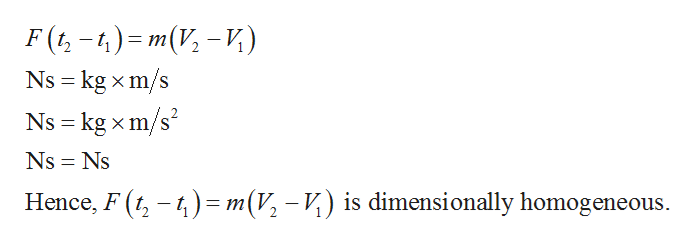# 6.4Which one of the following equations is dimensionally homogenous? Show yourproofF=maа.F = mb.F(t, - t) — т(V, -и)с.F = mV(V-V,)(12-)F = mе.whereF = force (N)m=mass (kg)a acceleration (m/s2)V velocity (m/s)R=radius (m)ttime (s)

Question
29 views

Engineering Fundamentals – An Introduction to Engineering

6th Edition – Saeed Moaveni

Chapter 6 Fundamental Dimensions and Units (Pages 157-200)

Question # 6.4 (Page 195)help_outlineImage Transcriptionclose6.4 Which one of the following equations is dimensionally homogenous? Show your proof F=ma а. F = m b. F(t, - t) — т(V, -и) с. F = mV (V-V,) (12-) F = m е. where F = force (N) m=mass (kg) a acceleration (m/s2) V velocity (m/s) R=radius (m) ttime (s) fullscreen
check_circle

star
star
star
star
star
1 Rating
Step 1

a)

Provide the dimensions in the equation F=ma as shown below.help_outlineImage TranscriptioncloseF = ma N-kg x m/s2 N N ma is dimensionally homogeneous Hence, F fullscreen
Step 2

b)

Provide the dimensions in the equation F=mV2/R as shown below.help_outlineImage TranscriptioncloseV 2 = m F (m/s)' N kg x N kg xm/s N N V2 is dimensionally homogeneous R Hence, F m fullscreen
Step 3

c)

Provide the dimensions in the equation F(t2-t1)=m(V2-V1) as shown bel...help_outlineImage TranscriptioncloseF(t-1) m(V -) s kg xm/s Ns kg xm/s X X Ns Ns Hence, F(4m(V, - V) is dimensionally homogeneous fullscreen

### Want to see the full answer?

See Solution

#### Want to see this answer and more?

Solutions are written by subject experts who are available 24/7. Questions are typically answered within 1 hour.*

See Solution
*Response times may vary by subject and question.
Tagged in

### Mechanics